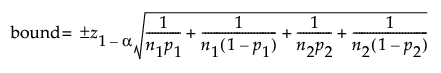Design of Experiments Guide > Sample Size Explorers > Confidence Interval Calculators > Margin of Error for Two Independent Sample Proportions
Publication date: 11/10/2021

## Margin of Error for Two Independent Sample Proportions

Use the Interval Explorer for Two Independent Sample Proportions to determine a sample size for a confidence interval for the difference in two proportions, for log relative risk, or for log odds. Select DOE > Sample Size Explorers > Confidence Intervals > Margin of Error for Two Independent Sample Proportions. Explore the trade offs between variability assumptions, sample size, significance, and the margin of error for your interval.

### Interval Explorer for Two Independent Sample Proportions Options

Set study assumptions and explore sample sizes using the radio buttons, text boxes, and sliders. The curve updates as you make changes to the settings. Alternatively, change settings by dragging the cross hairs on the curve or adjusting the values in the axis text boxes.

Interval Type

Bound

Specifies one side of the interval (upper or lower bound)

Interval

Specifies a two-sided interval.

Comparison Method

Difference in Proportions

Specifies a confidence interval for the difference in two proportions (p1 - p2).

Log Relative Risk

Specifies a confidence interval for the relative risk (p1/p2) on a log scale.

Log Odds

Specifies a confidence interval for the odds (p1/(1-p2)) on a log scale.

Fixed Parameters

Alpha

Specifies the confidence level, 1 - Alpha. The default alpha level is 0.05 for a 95% confidence interval.

Interval Parameters

Parameters that are inter-related and update as you make changes.

Group 1 Proportion

Specifies the assumed proportion for Group 1.

Group 2 Proportion

Specifies the assumed proportion for Group 2.

Group 1 Sample Size

Specifies the number of observations (runs, experimental units, or samples) needed for Group 1 in your experiment. Select Lock to lock the Group 1 sample size.

Group 2 Sample Size

Specifies the number of observations (runs, experimental units, or samples) needed for Group 2 in your experiment. Select Lock to lock the Group 2 sample size.

Total Sample Size

Specifies the total number of observations (runs, experimental units, or samples) needed for your experiment. The margin of error curve is based on total sample size. Select Lock to lock the total sample size.

Note: Adjusting the sample size for one group adjusts the total sample size unless the total sample size is locked. In that case, adjusting the sample size for one group adjust the sample size for the second group. Adjusting the total sample size adjusts the two groups proportionally, unless one group is locked. Use only one lock at a time.

Margin of Error

Specifies the half-width of the interval. With all other parameters fixed, margin of error decreases as sample size increases.

Bound Size

Specifies the bound of the interval. With all other parameters fixed, margin of error decreases as sample size increases.

Save Settings

Saves the current settings to the Saved Settings table. This enables you to save a set of alternative study plans. See Saved Settings in the Sample Size Explorers.

Make Data Collection Table

Creates a new data table that you can use for data collection.

### Statistical Details for the Two Independent Proportions Interval Explorer

The calculations for each interval type are based on normal approximations.

#### Difference in Proportions

For intervals about the difference in proportions the margin of error (MOE) is calculated as follows: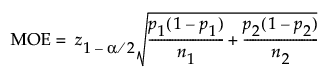The bounds are calculated as follows: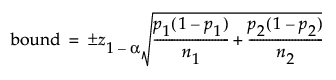#### Log Relative Risk

For the logarithm of the relative risk the margin of error (MOE) is calculated as follows: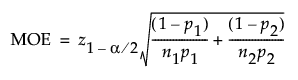The bounds are calculated as follows: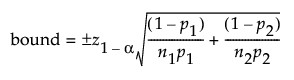#### Log Odds

For the logarithm of the odds ratio, the margin of error is calculated as follows: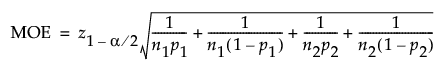The bounds are calculated as follows: